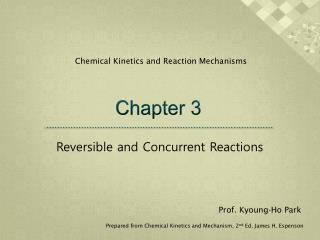DownloadDownload PresentationChapter 3

# Chapter 3

Télécharger la présentation## Chapter 3

- - - - - - - - - - - - - - - - - - - - - - - - - - - E N D - - - - - - - - - - - - - - - - - - - - - - - - - - -
##### Presentation Transcript

1. Chemical Kinetics and Reaction Mechanisms Chapter 3 Reversible and Concurrent Reactions Prof. Kyoung-Ho Park Prepared from Chemical Kinetics and Mechanism, 2nd Ed. James H. Espenson

2. 3.1 Reversible First-Order Reactions k1 A ↔ P k-1 = k1[A] – k-1[P] [A]0 + [P]0 = [A]e + [P]e = [A]t + [P]t k1[A]e = k-1[P]e [P]e/[A]e = K = k1/k-1 = (k1 + k-1) x ([A] – [A]e) ln{([A]t-[A]e)/([A]0-[A]e} = -(k1+k-1)t [A]t=[A]e+([A]0-[A]e)exp[-(k1+k-1)t] k1 = k-1= t1/2(e) = = Figure 3-1: A↔P k1 = 2.60 x 10-2 s-1t1/2 = 26.7 s k-1 = 4.10 x 10-2 s-1t1/2 = 10.3 s

3. 3.2 Opposing Reaction of Higher Order k1 A ↔ 2P k-1 k1 A ↔ P + Q k-1 k1 A + B ↔ P k-1 k1 A + B ↔ P + Q k-1 If [B]0 >> [A]0, plot of ln ([A]t –[A]e) versus timeis linear ke = k1[B] + k-1 k1 = k-1= Example of published result Co(edta)2- + Fe(CN)63- ↔ (edta)Co-NC-Fe(CN)55- Plot of ke versus [Co(edta)2-] is linear k1 = (3.36 ± 0.04) x 104 Lmol-1s-1 k-1 = 23.8 ± 2.8 s-1

4. k1 = 0.407 ± 0.0002 s-1 k-1 = 3.8 x 102 Lmol-1s-1 Figure 3-3 Triphenyl methyl system A ↔ 2P Figure 3-2 A plot of the first-order rate constant for equlibration.

5. 3.3 Concentration-Jump Methods for Opposing Reactions Useful method for the study of reversible reaction : -perturb the previous attained equilibrium ex) injecting component, diluting with solvent, change temperature A ↔ 2P = k1[A] ‒k-1[P]2 [A] = [A]e + δ [P] = [P]e - 2δ = k1([A]e + δ) – k-1([P]e - 2δ)2 equilibrium : k1[A]e = k-1[P]e2 = 4k-1δ2 – 4k-1[P]eδ – k1δk-1 = k1K-1 ln { δt / (α – 4K-1δt) = ln { δ0 / (α – 4K-1δ0} – k1αt δt = δ0αexp(-k1αt) / { α – 4K-1δ0[1 – exp(-k1αt)] }

6. 3.4 ExchangeReactions Method : NMR, EPR(electron paramagnetic resonance)

7. 3.5 Parallel and Concurrent Reactions

8. 3.6 Concurrent Reactions of Mixtures